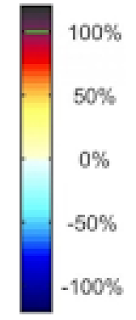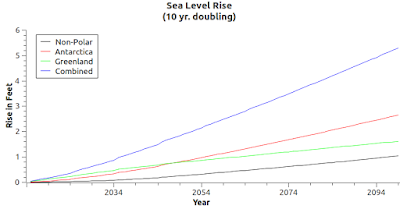## Wednesday, August 12, 2015

### The Question Is: How Much Acceleration Is Involved In SLR? - 8Fig. 1 How much?
Background

The question, as I will show in this post, is still what the title of this post proclaims (The Question Is: How Much Acceleration Is Involved In SLR?, 2, 3, 4, 5, 6, 7).

The graphic at Fig. 1 is from a Professor Mitrovica presentation on sea level change covered in a post where I pointed out that either sea level rise (SLR) or sea level fall (SLF) are equally catastrophic to the sea ports of current civilization (Peak Sea Level - 2).

Most important though, is that both SLR and SLF, in terms of when they may take place, are dependent on how much acceleration is going to take place in global warming.

Whether SLR or SLF happens to your port of choice is dependent first on when ice sheet meltwater or calving glaciers flow or slide into the sea, and that being the biggie, depends on acceleration of melt or calving caused by global warming (which depends on CO2 levels, which depends on the very dependable use of fossil fuels by Petroleum Civilization).

In other words, you can depend on either SLR or SLF coming to a sea port near you because of global warming induced climate change (brought to you by Oil-Qaeda: Oil-Qaeda: The Indictment).

And, you can bet that either SLR or SLF will be ugly (Why Sea Level Rise May Be The Greatest Threat To Civilization, 2, 3, 4, 5)

Sea Level Change By Way of Gravity?

In a recent post (The Gravity of Sea Level Change) I explained how Professor Mitrovica points out that the gravity of ice sheets becomes an important factor when considering whether your local sea port will be ruined by SLR or by SLF.

Does it really matter which one does a sea port in?

It does in terms of sea ports being the organs of current civilization, but it doesn't matter in terms of some other things (e.g. air travel).

Remember that gravity does not cause the ice sheets to melt, it causes the meltwater to conform akin to how it does tides every day.

Which is Worse SLR or SLF?

For an example situation, remember that SLR will come inland to damage, disrupt, or destroy homes, roads, and other infrastructure (e.g. ports).

But to the contrary, SLF will merely render homes, roads, and other infrastructure to be further away from the coast and ocean (that too may cause some problems).

Also, in the case of SLF, there would be fewer refugees in the sense that homes, cities, and towns along the coast would not become submarine structures, in whole or in part, as they will in the case of SLR.

The point to remember, in all cases, is that without sea ports current global trade based civilization cannot survive as we know it.

Changes are required (leave fossil fuels in the ground or suffer much worse) that are based on savvy as to both SLR and SLF.

"When And How Soon" Is the Cat's Meow!

"How much?" (Fig. 1) and "what kind?" (SLR or SLF) are secondary issues if they seem so far off in the future that everyone says "who cares?"

That is why "how soon?" or "how fast?" are the issues to keep in mind.

President Obama recently said "There is such a thing as being too late when it comes to climate change."

A Sea Level Change Software Model

I mentioned a while back that I am modifying my SLR software so that it will become an SLC software model.Fig. 2 Ice Sheet mass loss (10 yr doubling)

What that means is that it will calculate both SLR and SLF, along with the notion of when each one, hypothetically, will take place.

And where each one will take place.

The first order of business is to fuse the ice-mass-loss logic with the ice sheet melt logic, because they are intrinsically bound, even though they are not calculated in exactly the same way.

Notice that Fig. 2 and Fig. 3 have a similar look, because they are generated from the same data, but note that they do not have as much of a similar nature.Fig. 3 SLR (compare with Fig. 2)
I mean that in the sense that one dynamic takes place as a direct result of global warming induced climate change (ice melt = mass loss = gravity loss).

While the other (SLR or SLF) takes place afterwards, when the Earth takes the meltwater or ice bergs to their final destination to then become fluids in either an SLR environment or an SLF environment.

Nevertheless, the core structure of the software program is focused on the central issue, which is "when will the ice sheets melt and how fast will they melt?"

Conclusion

In closing, I will give one more preview or peek into the "remodel" work done so far on the SLC modelling software:
Force of gravity calculations:
-----------------------------
G = 6.67384 × 10-11 m3 kg-1 s-2
F = G((m1 * m2) / d2)
-----------------------------
For Antarctica:

when m1 = 2.95283e+07; m2 = 2.95283e+07; d = 100
F = 5.81906

when m1 = 2.95283e+07; m2 = 2.95283e+07; d = 200
F = 1.45476

when m1 = 2.95283e+07; m2 = 2.95283e+07; d = 300
F = 0.646562

when m1 = 2.95283e+07; m2 = 2.95283e+07; d = 400
F = 0.363691

when m1 = 2.95283e+07; m2 = 2.95283e+07; d = 500
F = 0.232762

when m1 = 2.95283e+07; m2 = 2.95283e+07; d = 600
F = 0.16164

when m1 = 2.95283e+07; m2 = 2.95283e+07; d = 700
F = 0.118756

when m1 = 2.95283e+07; m2 = 2.95283e+07; d = 800
F = 0.0909228

when m1 = 2.95283e+07; m2 = 2.95283e+07; d = 900
F = 0.0718402

when m1 = 2.95283e+07; m2 = 2.95283e+07; d = 1000
F = 0.0581906

-----------------------------
For Greenland:

when m1 = 2.62e+06; m2 = 2.62e+06; d = 100
F = 0.0458119

when m1 = 2.62e+06; m2 = 2.62e+06; d = 200
F = 0.011453

when m1 = 2.62e+06; m2 = 2.62e+06; d = 300
F = 0.00509021

when m1 = 2.62e+06; m2 = 2.62e+06; d = 400
F = 0.00286324

when m1 = 2.62e+06; m2 = 2.62e+06; d = 500
F = 0.00183248

when m1 = 2.62e+06; m2 = 2.62e+06; d = 600
F = 0.00127255

when m1 = 2.62e+06; m2 = 2.62e+06; d = 700
F = 0.000934937

when m1 = 2.62e+06; m2 = 2.62e+06; d = 800
F = 0.000715811

when m1 = 2.62e+06; m2 = 2.62e+06; d = 900
F = 0.000565579

when m1 = 2.62e+06; m2 = 2.62e+06; d = 1000
F = 0.000458119
Notice that as "d" (distance) increases the force of gravity "F" weakens considerably (by a factor of 100: 0.0458119 ÷ 0.000458119 = 100).

Also, notice that Antarctica's "F" is about 127 times more powerful than Greenland's "F" is (Antarctica's "F" @ 100 km = 5.81906; Greenland's "F" @ 100 km = 0.0458119; thus, 5.81906 ÷ 0.0458119 = 127).

That is very important when calculating and contemplating whether or not a sea port will "go down" due to SLR, or instead will "go down" because of SLF (due to Antarctica ice sheet melt, or Greenland ice sheet melt, or both).

Anyway, the SLC modelling software does not have very far to go before it is fully remodelled (a week or less).

The next post in this series is here, the previous post in this series is here.